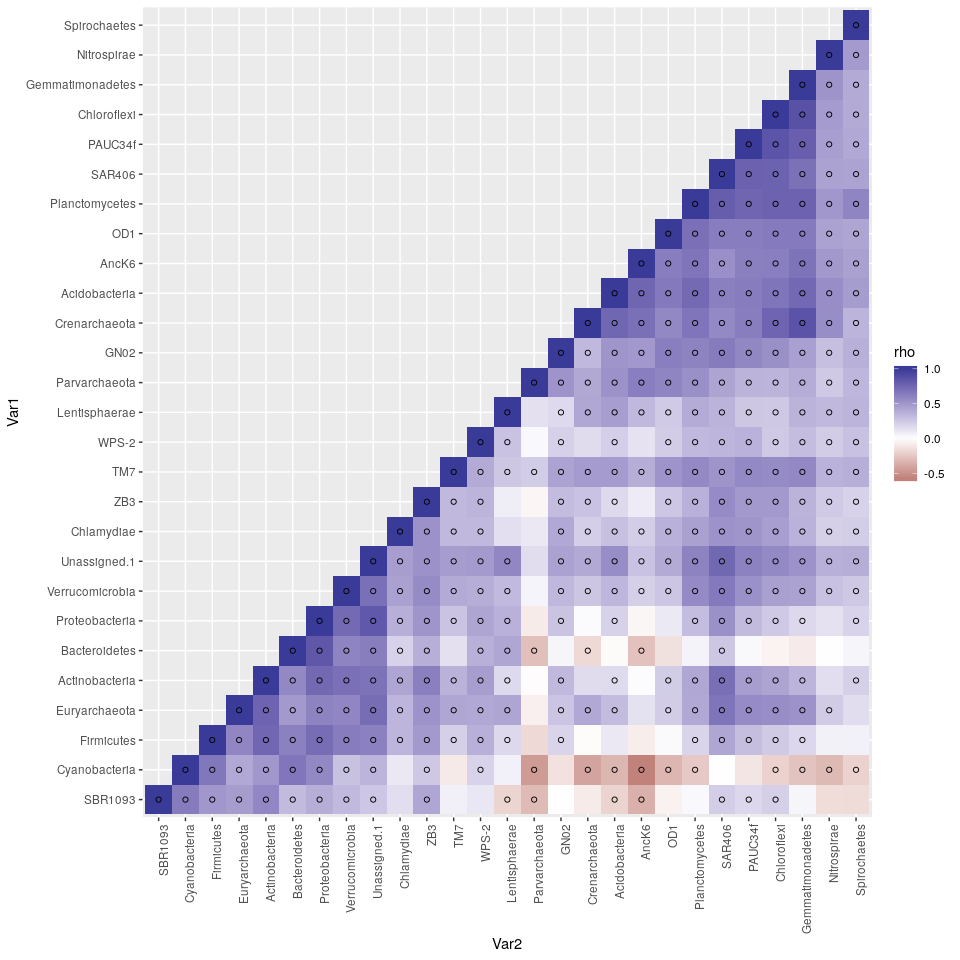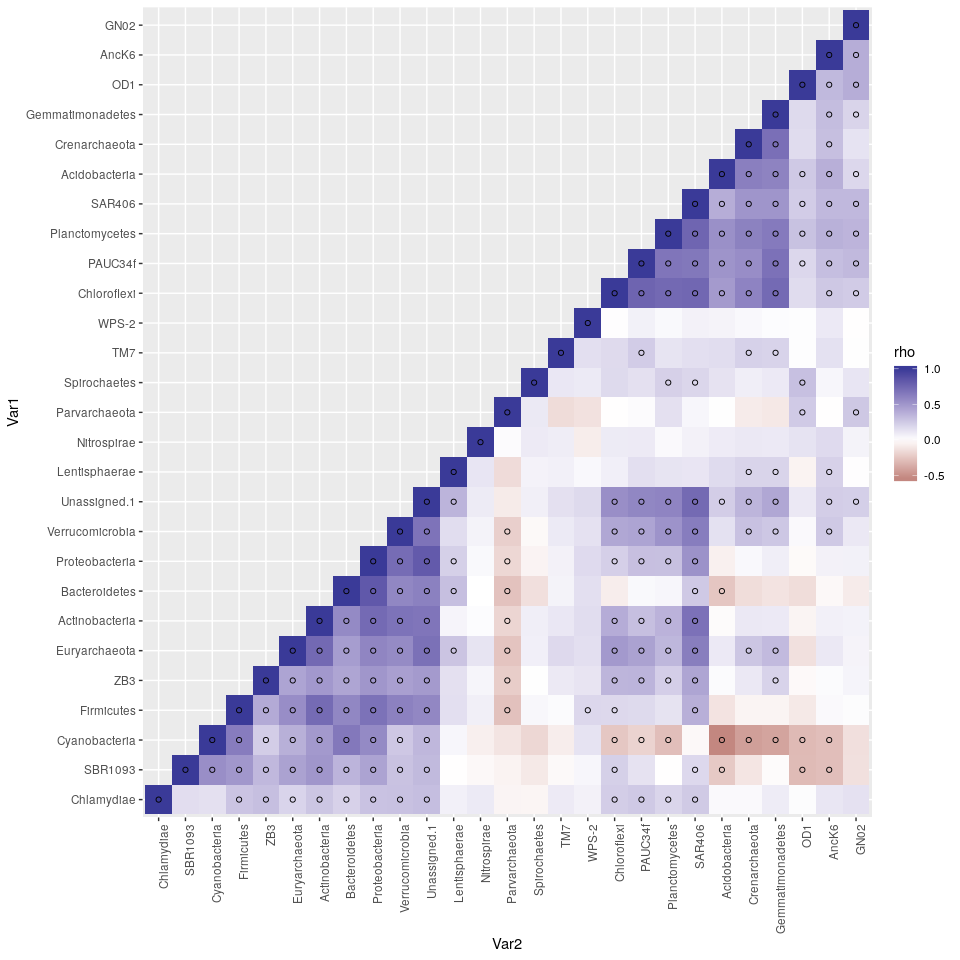In this lesson we will be generating a basic statistical association network form the Tara Oceans data that we can load into cytoscape.

We will generate three sets of statistics: Spearman correlations, spearman correlations on centered-log-ratio transformed data, and sparcc associations.

We will first apply a simple spearman correlation. A limitation of spearman correlations is that they don’t address the compositional nature of the data.

Compositional data are data where the different variables all sum to one. This paper by Lovell explains why this can be a problem.

Lovell D, Pawlowsky-Glahn V, Egozcue JJ, Marguerat S, Bähler J. Proportionality: A Valid Alternative to Correlation for Relative Data. PLOS Computational Biology. 2015 Mar 16;11(3):e1004075.

Essentially changes in the abundance of one variable makes all of the other values seem to be positively correlated with eachother.

To address this, we will first do a centered log ratio transform on our data, which convert them from compositions to ratios, and redo the spearman analyis.

Then we will use the sparcc algorithm that is specifically designed to handle these sorts of data.

# Installation

We’ll need a few packages for this tutorial. Lets load them in. If you don’t have any of these, you can install them using the `install.packages` function.

eg `install.packages("tidyverse")`

If you don’t have SpiecEasi, you can dowload it as follows. Note, you need to `install.packages("devtools")` if you haven’t ever done that before.

I commented the following lines out because I don’t want to re-run them every time I test this notebook out. Uncomment them and run if you need them.

``````#library(devtools)
#install_github("zdk123/SpiecEasi")``````

This took a while on my computer, you may want to get a cup of coffee while this runs.

``````library(tidyverse) # for plotting and wrangling data
library(SpiecEasi) # Has sparcc and also does clr transforms
library(otuSummary)
library(reshape2) # has the melt funct  ion, which I use to wrangle data
library(psych) # for calculating regular correlations with p values
pass <- function(x){x}``````

# Gathering data

First, lets dowload the Tara Oceans data from the internets. This data are read numbers of different phyla level bacterial and archaeal groups. The data were collected from all over the world. There are exciting environmental data collected as part of tara, but we will ignore those for now.

These data are read numbers of 16s genes but are from shotgun metagenomic sequencing.

``TaraPhyla <- read_tsv("https://www.ebi.ac.uk/metagenomics/api/v1/studies/MGYS00000410/pipelines/2.0/file/ERP001736_phylum_taxonomy_abundances_v2.0.tsv")``
``````Parsed with column specification:
cols(
.default = col_double(),
kingdom = [31mcol_character()[39m,
phylum = [31mcol_character()[39m
)
See spec(...) for full column specifications.``````

Please look over the new TaraPhyla object in your global environment. You can see that the first two columns are the Kindom and Phylum information for a bunch of bacterial and archaeal phyla. The rest of the columns are the number of reads associated with those phyla at each station. The station names are at the top.

# Pre-processing

While I’m a tidy-verse fanatic and like working with data in dataframes. If you want to correlate everything vs everything, its often necessary to transmute our data into a data frame.

``````TaraPhylaMtx0 <- TaraPhyla %>%
select(-kingdom)  %>% # remove kingdom column
filter(phylum != "unassigned") %>%
mutate(phylum = make.unique(phylum)) %>% # if you don't remove "unassigned, there are two phyla with this name and you have problems without this line
column_to_rownames(var = "phylum") %>% # turn phylum into row.names of data frame
as.matrix() %>% # transmogrify data.frame into matrix
t() %>% # transpose, so the OTUs are the columns and samples are the rows

pass

TaraPhylaMtx0[1:5, 1:5] # Take a look at part of the matrix``````
``````          Crenarchaeota Euryarchaeota Parvarchaeota AC1 AD3
ERR164407             0             0             0   0   0
ERR164408             1             0             0   0   0
ERR164409             0             0             0   0   0
ERR315858            38           149             0   0   0
ERR315859           178           478             0   0   0``````

It is is recommended to remove any species with fewer than two reads on average.

``````SpeciesToKeep <- apply(TaraPhylaMtx0, 2, mean) > 2

TaraPhylaMtx <- TaraPhylaMtx0[,SpeciesToKeep]``````

Also Also, lets normalize evertying to total read depth.

``````TaraPhylaMtxRA <- TaraPhylaMtx %>% sweep(2, colSums(.), "/") # RA for relative abundance
TaraPhylaMtxRA[1:5, 1:5]``````
``````          Crenarchaeota Euryarchaeota Parvarchaeota Acidobacteria Actinobacteria
ERR164407  0.000000e+00  0.0000000000             0  0.000000e+00   1.330922e-05
ERR164408  2.180074e-06  0.0000000000             0  0.000000e+00   2.994575e-05
ERR164409  0.000000e+00  0.0000000000             0  0.000000e+00   9.981916e-06
ERR315858  8.284282e-05  0.0003854302             0  3.341241e-05   1.620398e-03
ERR315859  3.880532e-04  0.0012364808             0  3.341241e-05   4.124195e-03``````

# Simple correlation analysis

The simplist way to look for associations in data is through correlation analyis. There are some problems with applying correlations to this kind of data and we’ll come back to that. For now though, lets calculate the spearman correlations on the data

I’m using the corr.test function from the `psych` library, because it returns p values. I’m not adjusting for multiple comparasons up front. We’ll do that later.

``````#spearCor <- cor(TaraPhylaMtx, method = "spearman")
spearCorTest <- corr.test(TaraPhylaMtxRA, method = "spearman", adjust = "none")
spearCor <- spearCorTest\$r
spearP <- spearCorTest\$p``````

Now we have a matrix of spearman correlations and a matrix of p values.

## Processing the results

I’m using a solution from here to get a nice plot. http://www.sthda.com/english/wiki/ggplot2-quick-correlation-matrix-heatmap-r-software-and-data-visualization

``````## Helper functions
# Get lower triangle of the correlation matrix
get_lower_tri<-function(cormat){
cormat[upper.tri(cormat)] <- NA
return(cormat)
}
# Get upper triangle of the correlation matrix
get_upper_tri <- function(cormat){
cormat[lower.tri(cormat)]<- NA
return(cormat)
}

reorder_cormat <- function(cormat){
# Use correlation between variables as distance
dd <- as.dist((1-cormat)/2)
hc <- hclust(dd)
cormat <-cormat[hc\$order, hc\$order]
}

reorder_cor_and_p <- function(cormat, pmat){
dd <- as.dist((1-cormat)/2)
hc <- hclust(dd)
cormat <-cormat[hc\$order, hc\$order]
pmat <- pmat[hc\$order, hc\$order]
list(r = cormat, p = pmat)
}

## Make sure that the data are ordered so that more related species   are closer together.
## Also ensure that the p values and correlations are in the same order
reordered_all <- reorder_cor_and_p(spearCor, spearP)
reordered_spearCor <- reordered_all\$r
reordered_spearP <- reordered_all\$p

## Just take the upper triangle of the correlation matrix, reshape it into a data frame, and improve the names of the variables
spearCor_processed <- reordered_spearCor  %>% get_upper_tri() %>% reshape2::melt() %>% na.omit() %>% rename(rho = value)
spearP_processed <- reordered_spearP  %>% get_upper_tri() %>% reshape2::melt() %>% na.omit() %>% rename(p = value)

# join the correlation and pvalue data frames
spearRhoP <- left_join(spearCor_processed, spearP_processed, by = c("Var1", "Var2")) %>%
# calculate the false discovery rate to adjust for multiple p values
mutate(fdr = p.adjust(p, method = "BH"))

spearRhoP``````

So there’s are data that we will use later and plot.

## Plotting the results

``````
# Identify which pairs are "statistically significant, given our fdr threshold"
fdrThresh <- 0.01 # fdr threshold
spearOkP <- spearRhoP%>% filter(fdr < fdrThresh)

spearRhoP_plot <- spearRhoP %>% ggplot(aes(x = Var2, y = Var1, fill = rho)) + geom_tile() + scale_fill_gradient2() + theme(axis.text.x = element_text(angle = 90, hjust = 1)) + geom_point(data = spearOkP, shape = 1)
spearRhoP_plot``````The little circles indicate statistical significance.

# As above, but using Clr to adjust for compositionality

The centered log ratio converts relative abundance data to ratio data. Here every species is reported as the log of its ratio of the average (actually geometric average, rather than additive average) bacterium in that column

``````TaraPhylaMtxClr <- clr(TaraPhylaMtx)
``````

Then we just do spearman correlation on the clr transformed data.

``````# spearCorClr <- stats::cor(TaraPhylaMtxClr, method = "spearman")

spearCorTestClr <- corr.test(TaraPhylaMtxClr, method = "spearman", adjust = "none")
spearCorClr <- spearCorTestClr\$r
spearPClr <- spearCorTestClr\$p``````

## Processing

``````reordered_all_Clr <- reorder_cor_and_p(spearCorClr, spearPClr)
reordered_spearCor_Clr <- reordered_all_Clr\$r
reordered_spearP_Clr <- reordered_all_Clr\$p

spearCor_processed_Clr <- reordered_spearCor_Clr  %>% get_upper_tri() %>% reshape2::melt() %>% na.omit() %>% rename(rho = value)
spearP_processed_Clr <- reordered_spearP_Clr  %>% get_upper_tri() %>% reshape2::melt() %>% na.omit() %>% rename(p = value)

# join the two data frames

spearRhoP_Clr <- left_join(spearCor_processed_Clr, spearP_processed_Clr, by = c("Var1", "Var2")) %>%
# # remove self correlations
# filter(Var1 != Var2) %>%
# calculate the false discovery rate to adjust for multiple p values
mutate(fdr = p.adjust(p, method = "BH"))``````

## Plotting

``````
spearOkP_Clr <- spearRhoP_Clr%>% filter(fdr < fdrThresh)

spearRhoPClr_plot <- spearRhoP_Clr %>% ggplot(aes(x = Var2, y = Var1, fill = rho)) + geom_tile() + scale_fill_gradient2() + theme(axis.text.x = element_text(angle = 90, hjust = 1)) + geom_point(data = spearOkP_Clr, shape = 1)

spearRhoPClr_plot``````# Analysisis with sparcc

At this point, I found this torial helpful. https://rachaellappan.github.io/16S-analysis/correlation-between-otus-with-sparcc.html

First, lets calculate the sparcc correlation values. At this point, we won’t have p-values yet.

``out <- sparcc(TaraPhylaMtx)``

Out is the output of sparcc. It contains a correlation and covariance matrix. Lets look at the first part of the correlation matrix.

``out\$Cor[1:5, 1:5]``
``````            [,1]       [,2]        [,3]       [,4]       [,5]
[1,]  1.00000000  0.1419885  0.03220802  0.5972360 -0.2126988
[2,]  0.14198851  1.0000000 -0.39138137 -0.2618167  0.4276109
[3,]  0.03220802 -0.3913814  1.00000000  0.1978761 -0.2784589
[4,]  0.59723601 -0.2618167  0.19787609  1.0000000 -0.4904111
[5,] -0.21269880  0.4276109 -0.27845885 -0.4904111  1.0000000``````

I’m kind of annoyed that it threw away my site names. Lets add them back

``````rownames(out\$Cor) <- colnames(TaraPhylaMtx)
colnames(out\$Cor) <- colnames(TaraPhylaMtx)
rownames(out\$Cov) <- colnames(TaraPhylaMtx)
colnames(out\$Cov) <- colnames(TaraPhylaMtx)
#cout <- as.data.frame(out\$Cor)
out\$Cor[1:5, 1:5]``````
``````               Crenarchaeota Euryarchaeota Parvarchaeota Acidobacteria Actinobacteria
Crenarchaeota     1.00000000     0.1419885    0.03220802     0.5972360     -0.2126988
Euryarchaeota     0.14198851     1.0000000   -0.39138137    -0.2618167      0.4276109
Parvarchaeota     0.03220802    -0.3913814    1.00000000     0.1978761     -0.2784589
Acidobacteria     0.59723601    -0.2618167    0.19787609     1.0000000     -0.4904111
Actinobacteria   -0.21269880     0.4276109   -0.27845885    -0.4904111      1.0000000``````

Better.

## Plotting Sparcc correlation

``````plotableSparcc <- out\$Cor %>% reorder_cormat %>% get_upper_tri() %>% reshape2::melt() %>% na.omit()

Sparcc_plot <- plotableSparcc %>% ggplot(aes(x = Var2, y = Var1, fill = value)) + geom_tile() + scale_fill_gradient2() + theme(axis.text.x = element_text(angle = 90, hjust = 1))
Sparcc_plot``````